﻿ 基于Kinect的健身动作识别与评价 Fitness Movement Recognition and Evaluation Based on Kinect

Computer Science and Application
Vol.08 No.07(2018), Article ID:26108,12 pages
10.12677/CSA.2018.87125

Fitness Movement Recognition and Evaluation Based on Kinect

Yi Wang, Xiaowen Zhu, Chengzhang Qu*

College of Information Engineering, Wuhan Business University, Wuhan Hubei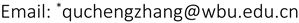Received: Jul. 5th, 2018; accepted: Jul. 20th, 2018; published: Jul. 27th, 2018ABSTRACT

As health issue enrolling a more important role in daily life, sports and fitness movements are accepted increasingly by the majority of people. How to exercise more effectively and reduce physical injuries reasonably is one of the hottest researches in the current scientific fitness movement. In this paper, we use Kinect to collect fitness movement data, then automatically classify the movements, and compare them with standard movements to calculate the degree of vulnerability. Specifically, we collect and preprocess the skeleton data of person’s movements first, and then we extract the feature and normalize the weight to form a representative feature among all movements. Second, we classify all the movements by little tuned KNN algorithm, and form the movement’s template model by similarity calculation. Third, we evaluate the movements based on the identified template and the injury model to guide the performance of movement. The experiments show the features we extract for all movements can represent the movement well that only little tuned KNN algorithm can get a competitive recognition result. We has compared neural net algorithm and KNN that the former is less generalized than the latter. The score and suggestion of fitness movement can reduce sports injuries and improve the enjoyment of fitness sports.

Keywords:KNN, Static Action Classification, Skeleton Data, Standardized Score1. 引言

KNN算法能够有效分类静态动作，神经网络具有较高的自学习和自适应能力。评分方法基于模板数据和测试数据特征向量的角度差，对一般常见健身动作可评分。

2. 相关技术

2.1. 基于Kinect的骨架特征选取

Kinect设备是由微软公司研发，能够在不使用控制器的情况下进行人机交互。在硬件上，从左往右依次是红外线发射器、RGB彩色摄影机和红外线摄影机。中间的镜头用来采集彩色图像，两边的镜头则用来采集深度数据。

2.2. 动作分类与评分方法

2.2.1. 动作分类

KNN (K最近邻，k-Nearest Neighbor)算法，即一个测试数据在特征空间中到模板的距离，在距离最小的K个数据中，属于某一类的数量最多，测试数据也属于此类别，并具有此类模板具有的特性。KNN算法结合其他领域的方法，在众多方向均有应用     。

${D}_{l}=sqrt\left\{{\left[{C}_{\text{test}}\left(1,v\right)-{C}_{\text{model}}\left(u,v\right)\right]}^{2}\cdot w\right\}$ (1)

2.2.2. 动作评分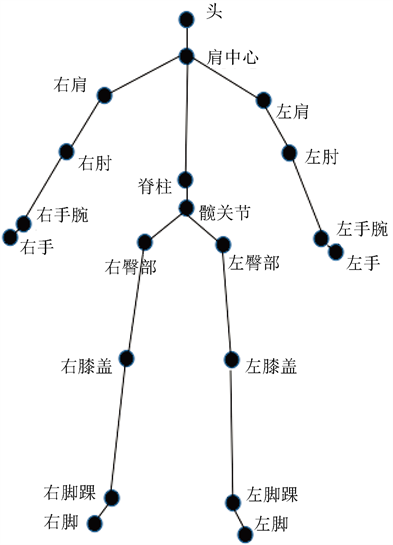Figure 1. Skeleton joint of human

1) 提出的静态姿势识别准确度计算公式( ${\alpha }_{\mathrm{max}}$ 为最大的 ${\alpha }_{i}$ )

$S=\left\{\begin{array}{l}f\left({\alpha }_{\mathrm{max}}\right)\cdot \left[\left({Z}_{st}-Z\right)\cdot \frac{100-{S}_{st}}{{Z}_{st}}+{Z}_{st}\right]\text{\hspace{0.17em}}\text{\hspace{0.17em}}\text{\hspace{0.17em}}\text{\hspace{0.17em}}\text{\hspace{0.17em}}\text{\hspace{0.17em}}0\le Z\le {Z}_{st}\\ 0\text{\hspace{0.17em}}\text{\hspace{0.17em}}\text{\hspace{0.17em}}\text{\hspace{0.17em}}\text{\hspace{0.17em}}\text{\hspace{0.17em}}\text{\hspace{0.17em}}\text{\hspace{0.17em}}\text{\hspace{0.17em}}\text{\hspace{0.17em}}\text{\hspace{0.17em}}\text{\hspace{0.17em}}\text{\hspace{0.17em}}\text{\hspace{0.17em}}\text{\hspace{0.17em}}\text{\hspace{0.17em}}\text{\hspace{0.17em}}\text{\hspace{0.17em}}\text{\hspace{0.17em}}\text{\hspace{0.17em}}\text{\hspace{0.17em}}\text{\hspace{0.17em}}\text{\hspace{0.17em}}\text{\hspace{0.17em}}\text{\hspace{0.17em}}\text{\hspace{0.17em}}\text{\hspace{0.17em}}\text{\hspace{0.17em}}\text{\hspace{0.17em}}\text{\hspace{0.17em}}\text{\hspace{0.17em}}\text{\hspace{0.17em}}\text{\hspace{0.17em}}\text{\hspace{0.17em}}\text{\hspace{0.17em}}\text{\hspace{0.17em}}\text{\hspace{0.17em}}\text{\hspace{0.17em}}\text{\hspace{0.17em}}\text{\hspace{0.17em}}\text{\hspace{0.17em}}\text{\hspace{0.17em}}\text{\hspace{0.17em}}\text{\hspace{0.17em}}\text{\hspace{0.17em}}\text{\hspace{0.17em}}\text{\hspace{0.17em}}\text{\hspace{0.17em}}\text{\hspace{0.17em}}\text{\hspace{0.17em}}\text{\hspace{0.17em}}\text{\hspace{0.17em}}\text{\hspace{0.17em}}\text{\hspace{0.17em}}\text{\hspace{0.17em}}\text{\hspace{0.17em}}\text{\hspace{0.17em}}\text{\hspace{0.17em}}Z\ge {Z}_{st}\end{array}$ (2)

2) 设置肢体偏移极限函数作为惩罚因子(M为预设肢体偏移最大角度阈值)

$f\left({\alpha }_{\mathrm{max}}\right)=\left\{\begin{array}{l}1-\frac{0.3}{{M}^{2}}\cdot {\alpha }_{\mathrm{max}}^{2}\text{\hspace{0.17em}}\text{\hspace{0.17em}}\text{\hspace{0.17em}}\text{\hspace{0.17em}}\text{\hspace{0.17em}}0\le {\alpha }_{\mathrm{max}}\le \sqrt{10/3}M\\ 0\text{\hspace{0.17em}}\text{\hspace{0.17em}}\text{\hspace{0.17em}}\text{\hspace{0.17em}}\text{\hspace{0.17em}}\text{\hspace{0.17em}}\text{\hspace{0.17em}}\text{\hspace{0.17em}}\text{\hspace{0.17em}}\text{\hspace{0.17em}}\text{\hspace{0.17em}}\text{\hspace{0.17em}}\text{\hspace{0.17em}}\text{\hspace{0.17em}}\text{\hspace{0.17em}}\text{\hspace{0.17em}}\text{\hspace{0.17em}}\text{\hspace{0.17em}}\text{\hspace{0.17em}}\text{\hspace{0.17em}}\text{\hspace{0.17em}}\text{\hspace{0.17em}}{\alpha }_{\mathrm{max}}\ge \sqrt{10/3}M\end{array}$ (3)

3. 静态动作识别与评分

3.1. 测试及模板数据获取

6种健身动作的镜面示范，如图3所示。

3.2. 特征值选取及分类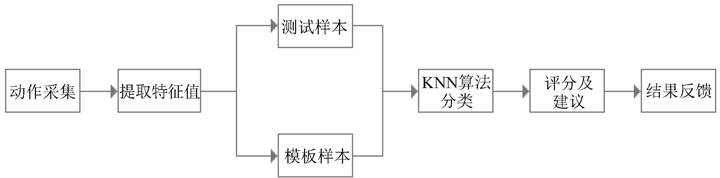Figure 2. Static fitness action classification and grading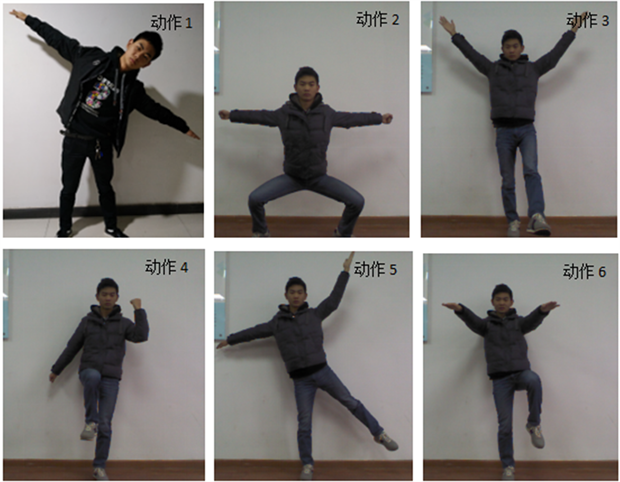Figure 3. Six fitness movements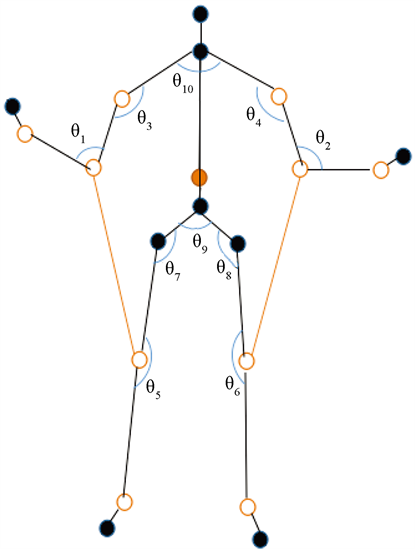Figure 4. Connection angle and distance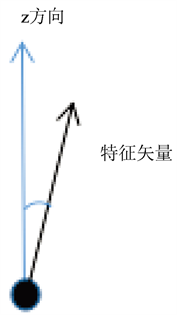Figure 5. Z axis Angle diagram of spine and standing foot

$n=\left({x}_{2}-{x}_{1},{y}_{2}-{y}_{1},{z}_{2}-{z}_{1}\right)$ (4)

$\text{cos}\theta =\frac{{x}_{WB}\cdot {x}_{SB}+{y}_{WB}\cdot {y}_{SB}+{z}_{WB}\cdot {z}_{SB}}{\sqrt{{x}_{WB}^{2}+{y}_{WB}^{2}+{z}_{WB}^{2}}\cdot \sqrt{{x}_{SB}^{2}+{y}_{SB}^{2}+{z}_{SB}^{2}}},\text{\hspace{0.17em}}\text{\hspace{0.17em}}\theta \in \left(0,{180}^{\circ }\right)$ (5)

1) 计算各个特征角度值之和(第l个特征角度 ${\theta }_{l}$ )

${\theta }_{\text{sum}}=\sum _{l=0}^{l=k}{\theta }_{l}$ (6)

2) 对 ${\alpha }_{l}$ 赋不同权重 ${w}_{l}$ ，减少模板与测试动作中角度差异性带来的误差

${w}_{i}=\frac{1-{\text{e}}^{-\frac{{\theta }_{l}}{{\theta }_{\text{sum}}}}}{\sum _{l=0}^{l=k}1-{\text{e}}^{-\frac{{\theta }_{l}}{{\theta }_{\text{sum}}}}}$ (7)

3) 得到归一化的角度值

$\mathrm{cos}{\theta }_{l}=\sum _{l=0}^{l=k}{\theta }_{l}\cdot {w}_{l}$ (8)

${d}_{l}=\sqrt{{\left({x}_{2}-{x}_{1}\right)}^{2}+{\left({y}_{2}-{y}_{1}\right)}^{2}+{\left({z}_{2}-{z}_{1}\right)}^{2}}$ (9)

$C=\left\{{d}_{1},{d}_{2},\cdots ,{d}_{12},\text{cos}{{\theta }^{\prime }}_{1},\text{cos}{{\theta }^{\prime }}_{2},\cdots ,\text{cos}{{\theta }^{\prime }}_{12}\right\}$ (10)

${C}_{\text{test}}$ 测试数据集中某一测试数据， ${C}_{\text{ave-model}}$ 模板数据的均值模板。

$D=sqrt\left\{{\left[{C}_{\text{test}}-{C}_{\text{ave-model}}\right]}^{2}\cdot w\right\}$ (11)

3.3. 评分方法

$f=\left\{\begin{array}{l}0\text{\hspace{0.17em}}\text{\hspace{0.17em}}\text{\hspace{0.17em}}\text{\hspace{0.17em}}\text{\hspace{0.17em}}\text{\hspace{0.17em}}\text{\hspace{0.17em}}\text{\hspace{0.17em}}\text{\hspace{0.17em}}\text{\hspace{0.17em}}\text{\hspace{0.17em}}\text{\hspace{0.17em}}\text{\hspace{0.17em}}\text{\hspace{0.17em}}\text{\hspace{0.17em}}\text{\hspace{0.17em}}\text{\hspace{0.17em}}\text{\hspace{0.17em}}\text{\hspace{0.17em}}\text{\hspace{0.17em}}\text{\hspace{0.17em}}\text{\hspace{0.17em}}\text{\hspace{0.17em}}\text{\hspace{0.17em}}\text{\hspace{0.17em}}\text{\hspace{0.17em}}\text{\hspace{0.17em}}\text{\hspace{0.17em}}\text{\hspace{0.17em}}\text{\hspace{0.17em}}\text{\hspace{0.17em}}\text{\hspace{0.17em}}\text{\hspace{0.17em}}\text{\hspace{0.17em}}\text{\hspace{0.17em}}\text{\hspace{0.17em}}\text{\hspace{0.17em}}\text{\hspace{0.17em}}\text{\hspace{0.17em}}\text{\hspace{0.17em}}\text{\hspace{0.17em}}\text{\hspace{0.17em}}\text{\hspace{0.17em}}\text{\hspace{0.17em}}\text{\hspace{0.17em}}\text{\hspace{0.17em}}\text{\hspace{0.17em}}\text{\hspace{0.17em}}\text{\hspace{0.17em}}\text{\hspace{0.17em}}\text{\hspace{0.17em}}\text{\hspace{0.17em}}\text{\hspace{0.17em}}\text{\hspace{0.17em}}\text{\hspace{0.17em}}\text{\hspace{0.17em}}\text{\hspace{0.17em}}\text{\hspace{0.17em}}\text{\hspace{0.17em}}\theta <{\beta }_{1}\cup \theta >{\beta }_{2}\\ \left(\frac{1}{\mathrm{cos}{\alpha }_{1}-\mathrm{cos}{\beta }_{1}}\right)\cdot \mathrm{cos}\theta +\frac{\mathrm{cos}{\beta }_{1}}{\mathrm{cos}{\beta }_{1}-\mathrm{cos}{\alpha }_{1}}\text{\hspace{0.17em}}\text{\hspace{0.17em}}\text{\hspace{0.17em}}\text{\hspace{0.17em}}\text{\hspace{0.17em}}\text{\hspace{0.17em}}\text{\hspace{0.17em}}{\beta }_{1}<\theta <{\alpha }_{1}\\ 1\text{\hspace{0.17em}}\text{\hspace{0.17em}}\text{\hspace{0.17em}}\text{\hspace{0.17em}}\text{\hspace{0.17em}}\text{\hspace{0.17em}}\text{\hspace{0.17em}}\text{\hspace{0.17em}}\text{\hspace{0.17em}}\text{\hspace{0.17em}}\text{\hspace{0.17em}}\text{\hspace{0.17em}}\text{\hspace{0.17em}}\text{\hspace{0.17em}}\text{\hspace{0.17em}}\text{\hspace{0.17em}}\text{\hspace{0.17em}}\text{\hspace{0.17em}}\text{\hspace{0.17em}}\text{\hspace{0.17em}}\text{\hspace{0.17em}}\text{\hspace{0.17em}}\text{\hspace{0.17em}}\text{\hspace{0.17em}}\text{\hspace{0.17em}}\text{\hspace{0.17em}}\text{\hspace{0.17em}}\text{\hspace{0.17em}}\text{\hspace{0.17em}}\text{\hspace{0.17em}}\text{\hspace{0.17em}}\text{\hspace{0.17em}}\text{\hspace{0.17em}}\text{\hspace{0.17em}}\text{\hspace{0.17em}}\text{\hspace{0.17em}}\text{\hspace{0.17em}}\text{\hspace{0.17em}}\text{\hspace{0.17em}}\text{\hspace{0.17em}}\text{\hspace{0.17em}}\text{\hspace{0.17em}}\text{\hspace{0.17em}}\text{\hspace{0.17em}}\text{\hspace{0.17em}}\text{\hspace{0.17em}}\text{\hspace{0.17em}}\text{\hspace{0.17em}}\text{\hspace{0.17em}}\text{\hspace{0.17em}}\text{\hspace{0.17em}}\text{\hspace{0.17em}}\text{\hspace{0.17em}}\text{\hspace{0.17em}}\text{\hspace{0.17em}}\text{\hspace{0.17em}}\text{\hspace{0.17em}}\text{\hspace{0.17em}}\text{\hspace{0.17em}}\text{\hspace{0.17em}}\text{\hspace{0.17em}}\text{\hspace{0.17em}}\text{\hspace{0.17em}}{\alpha }_{1}<\theta <{\alpha }_{2}\\ \left(\frac{1}{\mathrm{cos}{\alpha }_{2}-\mathrm{cos}{\beta }_{2}}\right)\cdot \mathrm{cos}\theta +\frac{\mathrm{cos}{\beta }_{2}}{\mathrm{cos}{\beta }_{2}-\mathrm{cos}{\alpha }_{2}}\text{\hspace{0.17em}}\text{\hspace{0.17em}}\text{\hspace{0.17em}}\text{ }\text{ }\text{\hspace{0.17em}}\text{\hspace{0.17em}}{\alpha }_{2}<\theta <{\beta }_{2}\end{array}\text{\hspace{0.17em}}\text{\hspace{0.17em}}\text{\hspace{0.17em}}\theta \in \left[0,{180}^{\circ }\right],f\in \left[0,1\right]$ (12)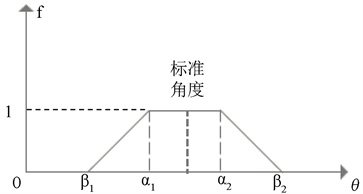Figure 6. Piecewise penalty function

$F=\mathrm{min}\left\{{f}_{1},{f}_{2},\cdots ,{f}_{11}\right\}$ (13)

${D}_{st}=\left\{1-sqrt\left[{\left(test-{D}_{\text{ave-model}}\right)}^{2}\cdot {W}_{i}\cdot F\right]\right\}\cdot 100$ (14)

4. 实验与结果分析

4.1. 实验步骤

1) 录入6种健身动作测试数据和标准模板数据；

2) 对数据进行预处理，并根据3.2.中公式提取健身动作特征值；

3) 根据公式11计算测试动作与分类模板动作特征值的距离差之和，得出分类结果；

4) 根据公式12计算易受伤惩罚函数的值；

5) 根据公式11计算测试动作与对应标准模板动作特征值的距离差之和，得出标准化程度；

6) 由公式12、13及14，综合计算标准化程度和易受伤程度；

7) 输出健身动作的评分和建议。

4.2. 实验设计与实验环境

4.3. 健身动作分类与对比实验

KNN能够准确找出错误动作与不标准动作，并识别错误角度给出提示。因此选择KNN算法进行分类，并在分类的基础上对健身动作进行评分。

4.4. 健身动作评分结果

4.5. 实验可修改方向

1) 由于录入数据样本时，Kinect对识别衣物的遮挡和动作中骨架的重叠会有影响，导致骨架点确立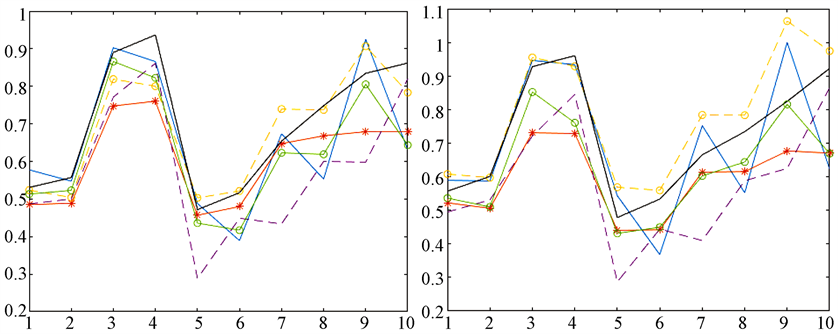Figure 7. Distance feature selection template compared with random template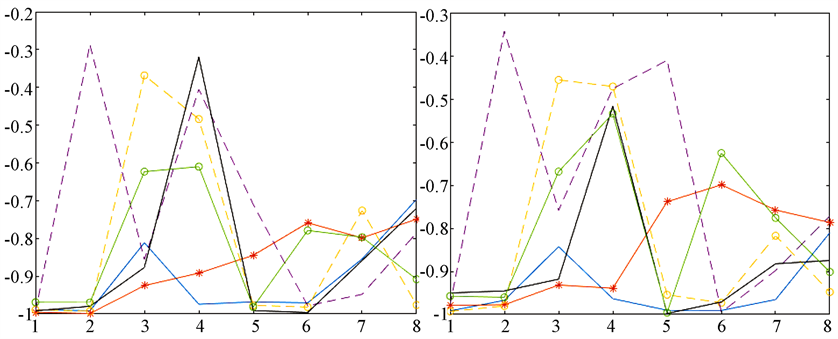Figure 8. 8 Angle features were selected from the template for random template comparison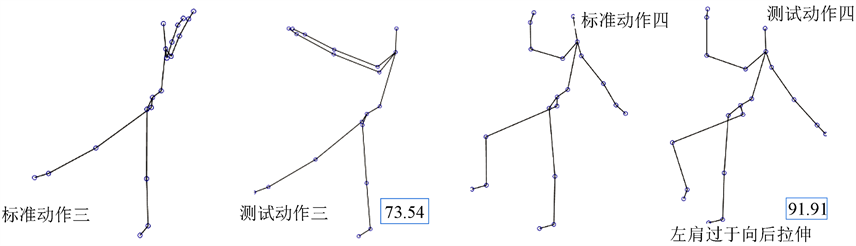Table 4. Comparison results between KNN algorithm and neural network

2) Kinect对体型不同的人骨架识别效果不同，对身材匀称、体重适中的健身者辨识度较高。对过高过瘦、或略微肥胖的健身者来说，收集数据时易产生数据异常  ；

3) 运动模板包括了标准的动作模板和分类模板，因未经过锻炼的人动作大多不标准。是否模板可以自适应调整，在收集到的动作模型中，挑选更好的模板进行替换；

4) 可以对评分类别、易受伤部位等进行细化，更为准确的判定运动受伤情况。

5. 结论

Fitness Movement Recognition and Evaluation Based on Kinect[J]. 计算机科学与应用, 2018, 08(07): 1134-1145. https://doi.org/10.12677/CSA.2018.87125

1. 1. 梁修容, 杨正益. 基于聚类和SVM的数据分类方法与实验研究[J]. 西南师范大学学报(自然科学版), 2018, 43(3): 91-96.

2. 2. 顾蒙蒙. 基于KNN算法的跑步姿态监测与识别[D]: [硕士学位论文]. 上海: 东华大学, 2017.

3. 3. 焦李成, 杨淑媛, 刘芳, 王士刚, 冯志玺. 神经网络七十年:回顾与展望[J]. 计算机学报, 2016, 39(0): 1697-1716.

4. 4. Liu, L., Wu, X., Wu, L., et al. (2012) Static Human Gesture Grading Based on Kinect. 5th Internation-al Congress on Image and Signal Processing, 1390-1393.

5. 5. 李红波, 李双生, 孙舶源. 基于Kinect骨骼数据的人体动作姿势识别方法[J]. 计算机工程与设计, 2016, 37(4): 969-975.

6. 6. 辛义忠, 邢志飞. 基于Kinect的人体动作识别方法[J]. 计算机工程与设计, 2016, 37(4): 1056-1061.

7. 7. 王枭. 基于改进KNN算法的二手房评估[D]: [硕士学位论文]. 哈尔滨: 哈尔滨商业大学, 2017.

8. 8. 李博, 郭琛, 任慧. 基于加权K近邻算法的抽象画图像情感分布预测[J]. 中国传媒大学学报(自然科学版), 2018, 25(1): 36-40.

9. 9. 黄贤英, 熊李媛, 刘英涛, 李沁东. 基于类别特征改进的KNN短文本分类算法[J]. 计算机工程与科学, 2018, 40(1): 148-154.

10. 10. 黄文明, 莫阳. 基于文本加权KNN算法的中文垃圾短信过滤[J]. 计算机工程, 2017, 43(3): 193-199.

11. 11. 邵丽. 基于关联规则的运动损伤风险评估仿真[J]. 现代电子技术, 2018(10): 172-174, 178.

12. 12. 李少聪, 马德, 李少琼. 基于大数据的运动损伤程度评估模型[J]. 现代电子技术, 2018, 41(6): 183-186.

13. 13. 李少聪, 马德, 李少琼. 运动损伤与运动强度的关系建模仿真分析[J]. 现代电子技术, 2017, 40(24): 37-39.

14. 14. 郑中华. 基于肢体动作的人体姿态识别研究[D]: [硕士学位论文]. 西安: 西安工业大学, 2015.

15. 15. 朱国刚, 曹林. 基于Kinect传感器骨骼信息的人体动作识别[J]. 计算机仿真, 2014, 31(12): 329-333, 345.

16. 16. 矫玮, 等. 运动损伤学双语教程[M]. 北京: 北京体育大学出版社, 35-42.

17. 17. 朱金宁, 李红娟. 青少年运动损伤与体质内因相关性分析[J]. 武汉体育学院学报, 2017, 51(4): 96-100.

NOTES

*通讯作者。